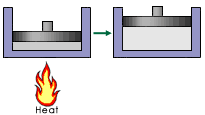# Thermodynamics Concept Test

## Problem 1

A piston is connected to a drive train that causes a car to move. The flame below is turned on, which heats up the gas causing it to expand.The signs of q and w for the gas in the cylinder are:

1. q +     w +
2. q +     w -
3. q -     w +
4. q -     w -

## Problem 2

Consider burning acetylene:
C2H2(g) + 3/2O2(g) <---> CO2 (g) + H2O(l) ΔH = -1301.1 kJ
What is ΔH for the reaction:
2CO2(g) + 2H2O(l) <---> 2C2H2(g) + 3O2(g)

1. ΔH = -2602.2 kJ
2. ΔH = -1301.1 kJ
3. ΔH = 1301.1 kJ
4. ΔH = 2602.2 kJ